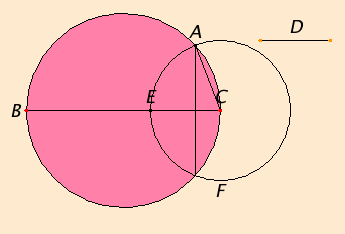# Proposition 1

To fit a straight line into a given circle equal to a given straight line which is not greater than the diameter of the circle.

Let ABC be the given circle, and D the given straight line not greater than the diameter of the circle

It is required to fit a straight line into the circle ABC equal to the straight line D.Draw a diameter BC of the circle ABC.

If BC equals D, then that which was proposed is done, for BC has been fitted into the circle ABC equal to the straight line D.

I.3

But, if BC is greater than D, make CE equal to D, describe the circle EAF with center C and radius CE, and join CA.

Then, since the point C is the center of the circle EAF, CA equals CE.

But CE equals D, therefore D also equals CA.

IV.Def.7

Therefore CA has been fitted into the given circle ABC equal to the given straight line D.

Q.E.F.

## Guide

The hypothesis that the line to be fitted into the circle is no longer than the diameter of the circle is certainly necessary, but Euclid did not show it was sufficient. That is sufficient to conclude the two circles actually meet at a point A is never demonstrated. This logical gap has appeared before in the Elements, for instance in Propositions I.1 and I.22.

This proposition is used in the proofs of IV.10, IV.16, and occasionally in Books X, XI, and XII.